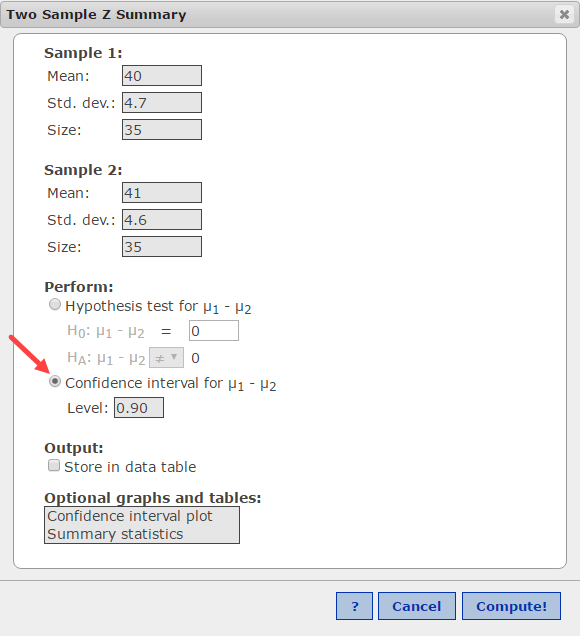# Hypothesis Test for Mean Difference using StatCrunch

I see a lot of students struggle with recognizing what a problem statement is asking them to do. Consider this problem:What do you get from that reading? I get:

• The phrase “Can the engineer support the claim…” tells me this is to be a hypothesis test.
• The second part of that sentence, “have different mean braking distances” indicates it is a test of the difference in means, µd = µ1 – µ2.
• That phrase also tells me the claim is “the means are different” which says the population means are not equal, µ1 ≠ µ2.
• Since the null hypothesis always is a form of equality, ≤, =, ≥, the null cannot be the claim, which makes the alternative the claim.
• The alternative hypothesis is the complement of the null, so the two hypotheses are:
Ho: µ1 = µ2 and Ha: µ1 ≠ µ2
• The math operator in the alternative always indicates the “tail” of the test. Here, it tells me the test is a two-tailed test.
• The fact that the standard deviations are σ’s, the population standard deviations, and not the sample standard deviations, s, tells me to run a z-test.[Note: some textbook authors say you can run the z-test without the population σ if n is > 30; other authors state you should always run the t-test if you do not have σ. As n increases beyond 30, the difference between the two tests becomes negligible but may be enough to trip you up if you are required to report answers in four decimal places. So, check with your textbook/instructor for the preference on this.]

I like to solve these types of problems using StatCrunch®.

First, find the critical values of z using the StatCrunch normal calculator: Stat > Calculators > Normal. I prefer to use the “Between” option for two-tailed tests and enter the confidence level, c = 1- alpha, in the probability box. Here, I entered 0.9 and clicked Compute.The resulting graph shows a red area under the curve which represents 0.9, which puts alpha/2 = 0.05 in each tail. The critical values of z are -1.645 and +1.645 and the rejection regions are z < -1.645 and z > +1.645.

Although you could solve for the test statistic manually usingand then use tables to find the p-value,

I prefer to use StatCrunch to do the entire test. Use the command sequence Stat > Z Stats > 2 Sample > With Summary.

In the dialog box that opens, enter the data for the two samples. Note: enter the population σ’s for the two samples and do not convert them to the sample standard error (standard deviation of the sampling distribution). Although you would do this if running the test “manually,” StatCrunch is set up to do this conversion for you.

Click Compute!The test statistic is -0.900, rounding to three decimals, and the p-value is 0.368. The test statistics does not fall in the rejection regions of <-1.645 or >+1.645, therefore the decision is to not reject the null hypothesis. That is the same result the p-value tells us since it is > alpha = 0.1.

Since the alternative is the claim, I would state my conclusion as:

At the 10% significance level, there is not enough evidence to support the claim that the mean braking distance for Type 1 tires is different than Type 2 tires.

Remember, you can quickly get the confidence interval around the mean difference by clicking on the Options button at the top left of the Output window, and click on Edit. Options > Edit. Then change select Confidence Interval for µ1µ2 and enter the confidence level desired. Then, click Compute!This site uses Akismet to reduce spam. Learn how your comment data is processed.